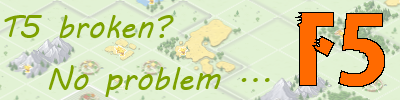Question about Tournament Square and Boots

• Hi,

Does TS and Boots work together ?

I mean If I had a TS lvl 20 (with 200% bonus speed) and Boots 100% speed ==> will it be 300% or just 200% ? based only TS ?

Thanks!

• They do work together, but combinations of ts + boots may yield ... weird results.

For instance, I have TS 4 + 30% boots. Let's take a target which is 25 fields away.

Without any boosts I would need 5 hours with pretorians (25 fields / 5 speed = 5 hours).

With TS only I need 4:42:51, which is logical: 20 fields with 5 speed, 5 fields with 5 * 1,4 = 7 speed equals 4,714285... hours equals 4:42:51. No surprises here.

With boots only I need 4:46:09, which is logical aswell: 20 fields with 5 speed, 5 fields with 5 * 1,3 = 6.5 speed equals 4,769230... hours equals 4:46:09. Also no surprises.

Ok. So far so good. But if I combine boots + TS, I have several options to calculate the speed for the 5 fields after the 20th. And none of them work out.

Logical options are:

- Boni work multiplicative = on top of each other (similar to oasis + goldboost production): Speed = 5 * 1,4 [for TS] * 1,3 [for boots], resulting in a speed of 9.1, which would turn out to be 4:32:58 in total

- Boni work additive = each affects the base speed (similar to oasis + mill/bakery): Speed = 5 * (100% + 40% [TS] + 30% [boots]), resulting in a speed of 8.5, which would turn out to be 4:35:17 in total

... but in reality it's 4:29:47. No idea what the fuck is happening in the calculation. If someone can enlighten me, I'd appreciate it.• The way the boots and TS stack is quite counter intuitive and results in troops moving faster than what you might expect them to when multiple bonuses are stacked.

Here's the quick rundown on how it works though. Instead of actually changing the speed the units move mid way trough the journey (which doesn't really make much sense since the units aren't physically moving on the map) the game instead calculates a new speed for the unit and then compares that to the distance to get the full travel time. The game does this by taking the base speed of the unit and then calculating what I will now call "boost factor" for the speed based on the TS level.

Lets use your numbers here for a distance of 25 tiles the pretorians travel 20 tiles at speed 5 and then 5 tiles at speed 7 if we combine these two for average speed over the entire journey we get a number that's roughly 5.303 speed or 1.0606 boost factor. You can find out the boost factor yourself with the following equation:

Total distance / ( 20 [for the first 20 tiles] + ( total distance - 20)/(1+speed buff))

Plug and chug here and we get a boost factor of

25/(20 +(25 - 20)/(1+0.4[TS multiplier]) = 1.06060606

1.06060606 * 5 = 5.3030303 [new speed]

Just to prove this provides the correct solution lets count the time here

25/5.3030303 = 4.71428572 hours -> 4h 42 minutes 51 seconds and bit which is what the game will display.

Now that the basics is out of the way the rest is quite simple. When there are multiple sources of speed buff the game simply multiplies up all the "boost factors" and then adds that to the real speed

In this case we need to calculate the boots boost factor so lets do that now and see if we get the right number

25 /(20+(25-20)/)1+0.3) = 1.0483871 boost factor

Total boost factor = 1.0483871 * 1.06060606 = 1.11192571

New speed = 5 * 1.11192571 = 5.55962855

Plug that to the distance and we get

25/5.55962855 = 4.49670329h -> 4h 29minutes 48seconds which is 1 second off of what the game actually shows which I believe is just due to some rounding here and there. Game probably uses bit fewer decimal places than I did in this to arrive to a speed just hair higher than I did.

While I personally believe the speed should work around simply multiplying the bonuses this is the way it works and essentially it has some light exponential curve you might not expect to be there on initial inspection.

Hope it helps• yes, thanks so much guys• Woah. Thanks, Curtain . This is ... the pure definition of counter-intuitive. Even though I thought about the "total boosted speed" (5.303 in the first example), since it's relevant for speed analysis, I probably wouldn't have come up with that calculation method at all. I assume, that standards for kingdom & account work similarly, just with a simpler "boost calculation"?• Be2-e4 Haven't tested those as defensive speed often isn't really relevant the same way boots + TS stacking is on offense but ye pretty sure they work the same way. If they do work like that you can get some serious exponential gains on your speed with cross map reinforcements with hero boots + pennant + TS• Yes, that's true. I'm just wondering, because we tried to figure out the pennant + ts combo for a while, while being confused a few months ago. xD